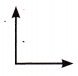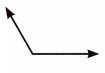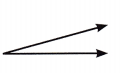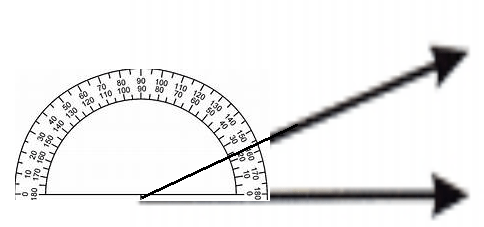# McGraw Hill Math Grade 4 Chapter 13 Lesson 2 Answer Key Measuring Angles

Practice the questions of McGraw Hill Math Grade 4 Answer Key PDF Chapter 13 Lesson 2 Measuring Angles to secure good marks & knowledge in the exams.

## McGraw-Hill Math Grade 4 Answer Key Chapter 13 Lesson 2 Measuring Angles

Measure

Use a protractor to measure each angle.

Question 1.90

Question 2.Explanation:
The measure of the angle is 120-degree.

Question 3.Explanation:
The measure of the angle is 20-degrees.

Question 4.Explanation:
The measure of the angle is 155-degrees.

Question 5.
What is the angle measure of a straight line?